Click to Chat

1800-1023-196

+91-120-4616500

CART 0

• 0

MY CART (5)

Use Coupon: CART20 and get 20% off on all online Study Material

ITEM
DETAILS
MRP
DISCOUNT
FINAL PRICE
Total Price: Rs.

There are no items in this cart.
Continue Shopping• Complete Physics Course - Class 11
• OFFERED PRICE: Rs. 2,968
• View Details

Motion of Projectile

Table of Content

Total Time of Flight

Horizontal Range

Maximum Height

Equation of Trajectory

Related Resources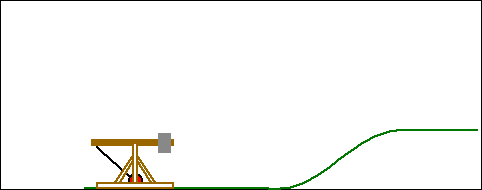Now we discuss some example of curved motion or two dimensional motion of constant acceleration such as the motion of constant acceleration such as the motion of a particle projected at certain angle with the horizontal in vertical x-y plane (this type of motion is called projectile motion). Air resistance to the motion of the body is to be assumed absent in this type of motion.

A body projected into the space and is no longer being propelled by fuel is called a projectile.

To analyze the projectile motion we use the following concept "Resolution of two dimensional motion into two one dimension motion" as discussed earlier. Hence it is easier to analyze the motion of projectile as composed of two simultaneous rectilinear motions which are independent of each other:

(a) Along the vertical y-axis with a uniform downward acceleration 'g' and

(b) Along the horizontal x-axis with a uniform velocity forward.

Consider a particle projected with an initial velocity u at an angle θ with the horizontal x-axis as shown in figure shown below. Velocity and accelerations can be resolved into two components:Velocity along x-axis = ux = u cos θ

Acceleration along x-axis ax = 0

Velocity along y-axis = uy = u sin θ

Acceleration along y-axis ay = -g

Here we use different equation of motions of one dimension derived earlier to get the different parameters.

…... (a)

…... (b)

v2 = v02 – 2g (y-y0)                      …... (c)

Total Time of Flight

When body returns to the same horizontal level, the resultant displacement in vertical y-direction is zero. Use equation b.

Therefore, 0 = (u sin θ) t - (½)gt2,

As t cannot equal to zero, then, total time of flight,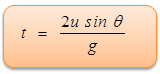Horizontal Range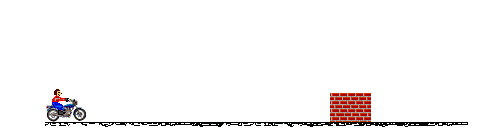Horizontal Range (OA=X)   = Horizontal velocity × Time of flight

= u cos θ × 2 u sin θ/g

So horizontal range,Maximum Height

At the highest point of the trajectory, vertical component of velocity is zero.

Therefore, 0 = (u sin θ)2 - 2g Hmax

So, maximum height would be,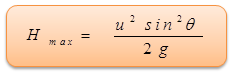Refer this video for better understanding about projectile motion:-

Equation of Trajectory

Assuming the point of projection as the origin of co-ordinates and horizontal direction as the x-axis and vertical direction as the y-axis. Let P (x, y) be the position of the particle at instant after t second.

Then x = u cos θ.t and y = u sin θ.t - 1/2 gt2

Eliminating 't' form the above equations, we get,

y = x tan θ - (gx2/2u2cos2θ)

This is the equation of trajectory which is a parabola (y = ax + bx2).

Refer this simulation for projectile motion:-

A ball is launched at an angle and lands at the same height it is launched from. A camera takes a picture of the flight every second on the same frame of film. The images are then moved down and to the right to show the independence of horizontal and vertical motion.

The speed of a projectile when it is at its greatest height is √2/5 times its speed at half the maximum height. Find out the angle of projection.

Solution :-

Let θ be the angle of projection and u its initial speed. Then maximum height will be,

H = u2sin2θ/2g

So, gH = u2sin2θ/2

Now, vH = u cosθ

Or, vH2 = u2 cos2θ                  …... (1)

vH/22 = u2-2g(H/2) = u2-gH

vH/22= u2-(u2sin2θ/2)             …...(2)

Now it is given that,

vH = [√2/5] vH/2

Or, vH2 = (2/5) vH/22

Substituting the values from equations (1) and (2), we get,

(u2 cos2θ) = 2/5 [u2-(u2sin2θ/2)]

Or, 5cos2θ = 2[1-(sin2θ/2)]

Or, 5(1-sin2θ) = 2-sin2θ

Or, sin2θ = ¾

Or, sinθ = (√3)/2, Or, θ = 60o

Thus from the above observation, we conclude that, the angle of projection would be 60o.

Problem 2:-

A gun moving at a speed of 30m/sec fires at an angle 30o with a velocity 150m/s relative to the gun. Find the distance between the gun and the projectile when projectile hits the ground. (g = 10 m/sec)

Solution :-

Vertical component of velocity = 150 sin 30o  = 75 m/sec

Horizontal component of velocity relative to gun = 150 cos 30o = 75√3 m/sec

Horizontal component of velocity relative to ground = 75√3 + 30 ≈ 160m/sec

Time of flight   = (275)/g = 15 s

Range of projectile = 160 × 15 = 2400 m

Distance moved by the gun and projectile = 2400 - 450 = 1950 m.

Horizontal Projection:-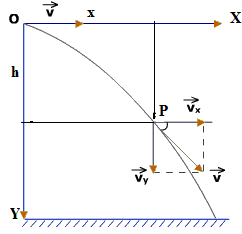Consider a particle projected horizontally with a velocity  from a point O as shown in side figure.

Assuming the point of projection O as the origin of coordinates and horizontal direction as the X-axis and vertical direction as Y-axis. Let P (x, y) be the position of the particle after t seconds.

So, x = horizontal distance covered in time t = ut.         ............... (1)

y = vertical distance covered in time t = ½gt2               ............... (2)

Eliminate t from equations (1) and (2) then we get,

y = (1/2)(g/u2) (x2)

This is the equation of parabola passing through the origin, with its vertex at the origin O. Hence the trajectory is a parabola.

Problem 3:-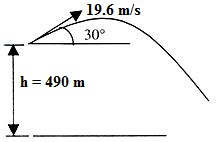A stone is thrown at a speed of 19.6 m/sec at an angle 30o above the horizontal from a tower of height 490 meter. Find the time during which the stone will be in air. Also find the distance from the foot of the tower to the point where stone hits the ground?

Solution :-

Let us consider the motion of stone in the horizontal and vertical directions separately.

(i) Vertical motion (downward direction negative) :

Initial vertical velocity y = 19.6 sin 30o

Acceleration a = g = -9.8 m/s2

Vertical distance covered = h = 490 m

Using, h = ut +  1/2gt2

We have, 490 = - 9.8t + (1/2) 9.8t2

100 = - 2t + t2           or     t2 - 2t - 100 = 0

t = 11.05 sec

(ii) Horizontal motion:

Initial horizontal velocity y = 19.6 sin 30o = 9.8 m/s

Hence distance from the foot of tower to the point where stone hits the ground

= Horizontal component × time of flight

= 19.6 cos 30o × 11.05 = 188 m

Projectile Motion on an inclined plane:-

Let the particle strike the plane at A so that OA is the range of the projectile on inclined plane. This initial velocity  can be resolved into two components:(i) u cos (α - β) along the plane

(ii) u sin (α - β) perpendicular to the plane.

The acceleration due to gravity g can be resolved into two components:

(i) g sin β parallel to the plane

(ii) g cos β perpendicular to the plane.

Time of Flight:-

Let t be the time taken by the particle to go from A to B. In this time the displacement of the projectile to the plane is zero.

Hence, 0 = u sin (α-β) t - ½g β t2

=> t = 2u sin(α-β)/gcosβ

Range:-

During time of flight, the horizontal velocity u cos α remains constant.

Hence, horizontal distance,

OB = (ucosα) t = 2u2sin(α-β)cosα/gcosβ

Now, OA = OB/cosβ = 2u2sin(α-β)cosα/gcosβ

The greatest distance of the projectile from the inclined plane is u2sin2 (α-β)/2gcosβ .

Problem 4:-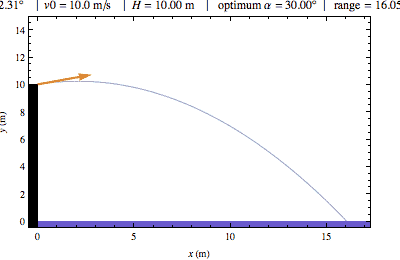A Particle is projected with a velocity 39.2 m/sec at an angle of 30o to an inclined plane (inclined at an angle of 45o to the horizontal). Find the range on the incline (a) when it is projected upward (b) when it is projected downward.

Solution :-

Time of flight will be same in both cases because the acceleration perpendicular to the plane is same. Therefore,

0 = 39.2 sin 30o t - (½) g cos 45o t2

Or, t = (2×39.2 sin 30)/(g cos 45) = 4√2  sec

(a) Range upward

= 39.2 cos 30o t - (½) g sin 30o t2

= 39.2 × √3/2 × 4√2- (1/2) × 9.8 × (1/2) × (4√2)2  = 113.7m

(b) Range downward

= 39.2 cos 30o × t + (½) g sin 30o t2

= 39.2 × √3/2 × 4√2  + (1/2) × 9.8 × (1/2) × (4√2)2  = 270.5m  Ans.

Motion down the plane:-Let the particle be thrown at a velocity v0 at angle ‘α’ with the horizontal as shown in figure.

v0  sin (α+β)T- 1/2 gcosβT2=0 [for y'=0]

=> T = (2v0 sin(α+β))/gcosβ

R = v0 cos(α+ β)T+ 1/2 g sin βT2= (v02)/g  [(sin(2α+β)+sinβ)/(1-sin2β)]

Since α is the variable and maximum value of sin function is 1, therefore for R to be maximum, sin (2α+β)=1

and Rmax (v02)/g [(1+sinβ)/(1-sin2β)]= (v02)/(g(1-sinβ)) down the plane.Every projectile experiences one single force and that is due to gravity only.

Horizontal velocity of a projectile remains the same throughout its flight (it may be zero also).

No projectile ever experiences any acceleration in the horizontal direction.

Vertical acceleration of every projectile is ‘-g’.

The path of projectile is parabolic except for those projected along vertical direction. In that case it is a straight line.

The horizontal and vertical motion of a projectile are independent of each other.Question 1:-

Which of the following is not a projectile:

(a) a bullet fired from a rifle                   (b) a bomb dropped from an aeroplane

(c) a cricket ball moving in space          (d) hydrogen balloon floating in air

Question 2:-A particle projected horizontally from the top of a tower clears range equal to the height of a tower. The path described is a part of a:

(a) circle                (b) ellipse

(c) hyperbola          (d) parabola

Question 3:-

A particle is thrown vertically upward. At its highest point, it has:

(a) an upward velocity                           (b) downward velocity

(c) an upward acceleration                     (d) a downward acceleration

Question 4:-

A particle is thrown vertically upward. Its velocity at half of the height is 10 m/s, then maximum height attained by it:

(a) 8 m                    (b) 20 m

(c) 10 m                  (d) 16 cm

Question 5:-

A body is projected horizontally from the top of a tower 19.6 meter high. It reaches the ground in:

(a) 1 sec                  (b) 2 sec

(c) 2.5 sec               (d) 5 secQ.1
Q.2
Q.3
Q.4
Q.5

b

d

d

c

b

Related Resources

You might like to refer Gravitation.

For getting an idea of the type of questions asked, refer the  Previous Year Question Papers.

To read more, Buy study materials of Kinematics comprising study notes, revision notes, video lectures, previous year solved questions etc. Also browse for more study materials on Physics here.### Course Features

• 101 Video Lectures
• Revision Notes
• Previous Year Papers
• Mind Map
• Study Planner
• NCERT Solutions
• Discussion Forum
• Test paper with Video Solution